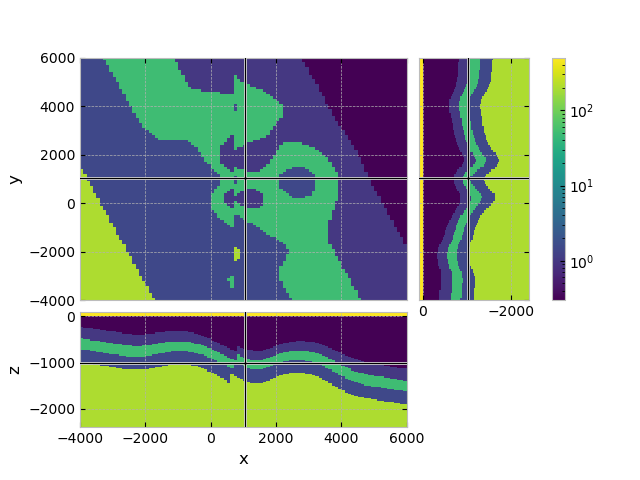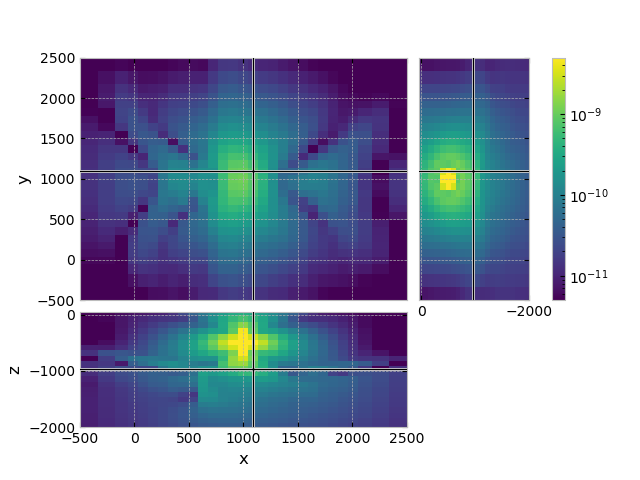# GemPy-I: Simple Fault Model#

This example uses GemPy to create a geological model as input to emg3d, utilizing discretize. Having it in discretize allows us also to plot it with PyVista.

The starting point is the simple_fault_model as used in Chapter 1.1 of the GemPy documentation. It is a nice, made-up model of a folded structure with a fault. Here we slightly modify it (convert it into a shallow marine setting), and create a resisistivity model out of the lithological model.

The result is what is referred to in other examples as model GemPy-I, a synthetic, shallow-marine resistivity model consisting of a folded structure with a fault. It is one of a few models created to be used in other examples.

Note

The original model (simple_fault_model) hosted on https://github.com/cgre-aachen/gempy_data is released under the LGPL-3.0 License.

```import os
import pooch
import emg3d
import matplotlib.pyplot as plt
from matplotlib.colors import LogNorm
plt.style.use('bmh')

# Adjust this path to a folder of your choice.
```

## Fetch the model#

Retrieve and load the pre-computed resistivity model.

```fname = "GemPy-I.h5"
pooch.retrieve(
'https://raw.github.com/emsig/data/2021-05-21/emg3d/models/'+fname,
'06f522a69c94dc02ca3da0ea4ca7b60f7a9c764cdcbf6699ef4155621d70b3bb',
fname=fname,
path=data_path,
)
fmodel = emg3d.load(data_path + fname)['model']
fgrid = fmodel.grid
```
```Data loaded from «/home/dtr/3-GitHub/emsig/emg3d-gallery/examples/download/GemPy-I.h5»
[emg3d v1.0.0rc3.dev5+g0cd9e09 (format 1.0) on 2021-05-21T14:06:32.551618].
```

## QC resistivity model#

```fgrid.plot_3d_slicer(
fmodel.property_x, zslice=-1000,
pcolor_opts={'norm': LogNorm(vmin=0.3, vmax=500)}
)
```## Compute some example CSEM data with it#

```# Source:  x-directed electric-source at (1000, 1000, -500)
src_coo = [1000, 1000, -500, 0, 0]
frequency = 1.0  # Hz

# Computational grid
grid = emg3d.construct_mesh(
frequency=frequency,
center=src_coo[:3],
properties=[0.3, 200, 1000],
domain=([0, 2000], [0, 2000], [-2000, 0]),
seasurface=0,
center_on_edge=False,
)
grid

# Get the computational model
model = fmodel.interpolate_to_grid(grid)

# Compute the response
efield = emg3d.solve_source(
model=model,
source=emg3d.TxElectricDipole(src_coo),
frequency=frequency,
verb=1,
)

# Plot the response
grid.plot_3d_slicer(
efield.fx.ravel('F'),
view='abs', v_type='Ex',
zslice=-1000,
xlim=(-500, 2500), ylim=(-500, 2500), zlim=(-2000, 50),
pcolor_opts={'norm': LogNorm(vmin=5e-12, vmax=5e-9)},
)
``````:: emg3d :: 7.9e-07; 2(9); 0:00:27; CONVERGED
```

## Reproduce the model#

Note

The following sections are about how to reproduce the model. For this you have to install `gempy`. The code example and the `GemPy-I.h5`-file used in the gallery were created on 2021-05-21 with `gempy=2.2.9` and `pandas=1.2.4`.

## Get and initiate the simple_fault_model#

Changes made to the original model (differences between the files simple_fault_model_*.csv and simple_fault_model_*_geophy.csv): Changed the stratigraphic unit names, and moved the model 2 km down.

Instead of reading a csv-file we could also initiate an empty instance and then add points and orientations after that by, e.g., providing numpy arrays.

```import gempy as gempy
import numpy as np

# Initiate a model
geo_model = gempy.create_model('GemPy-I')

# Location of data files.
data_url = 'https://raw.githubusercontent.com/cgre-aachen/gempy_data/'
data_url += 'master/data/input_data/tut_chapter1/'

# Importing the data from CSV-files and setting extent and resolution
# This is a regular grid, mainly for plotting purposes
gempy.init_data(
geo_model,
[0, 2000., 0, 2000., -2000, 40.], [50, 50, 51],
path_o=data_url+"simple_fault_model_orientations_geophy.csv",
path_i=data_url+"simple_fault_model_points_geophy.csv",
)
```

## Initiate the stratigraphies and faults, and add an air layer#

```# Add an air-layer: Horizontal layer at z=0m
geo_model.add_surface_points(0, 0, 0, 'air')
geo_model.add_surface_points(0, 0, 0, 'air')
geo_model.add_orientations(0, 0, 0, 'air', [0, 0, 1])

# Add Series for the air layer; this series will not be cut by the fault
geo_model.modify_order_series(2, 'Air_Series')
gempy.map_series_to_surfaces(geo_model, {'Air_Series': 'air'})

# Map the different series
gempy.map_series_to_surfaces(
geo_model,
{
"Fault_Series": 'fault',
"Air_Series": ('air'),
"Strat_Series": ('seawater', 'overburden', 'target',
'underburden', 'basement')
},
remove_unused_series=True
)

geo_model.rename_series({'Main_Fault': 'Fault_Series'})

# Set which series the fault series is cutting
geo_model.set_is_fault('Fault_Series')
geo_model.faults.faults_relations_df
```

## Compute the model with GemPy#

```# Set the interpolator.
gempy.set_interpolator(
geo_model,
compile_theano=True,
theano_optimizer='fast_compile',
verbose=[]
)

# Compute it.
sol = gempy.compute_model(geo_model, compute_mesh=True)

# Plot lithologies (colour-code corresponds to lithologies)
_ = gempy.plot_2d(geo_model, cell_number=25, direction='y',
show_data=True)
```

## Get id’s for a discretize mesh#

We could define the resistivities before, but currently it is difficult for GemPy to interpolate for something like resistivities with a very wide range of values (several orders of magnitudes). So we can simply map it here to the `id` (Note: GemPy does not do interpolation for cells which lie in different stratigraphies, so the id is always in integer).

```# First we create a detailed discretize-mesh to store the resistivity
# model and use it in other examples as well.
hxy = np.ones(100)*100
hz = np.ones(100)*25
fgrid = emg3d.TensorMesh([hxy, hxy, hz], origin=(-4000, -4000, -2400))

# Get the solution at cell centers of our grid.
sol = gempy.compute_model(geo_model, at=fgrid.gridCC)

# Show the surfaces.
geo_model.surfaces
```

## Replace id’s by resistivities#

```# Now, we convert the id's to resistivities
res = sol.custom[0, :fgrid.n_cells]

res[res == 1] = 1e8  # air
# id=2 is the fault
res[np.round(res) == 3] = 0.3  # sea water
res[np.round(res) == 4] = 1.0  # overburden
res[np.round(res) == 5] = 50   # resistive layer
res[np.round(res) == 6] = 1.5  # underburden
res[np.round(res) == 7] = 200  # resistive basement

# Create an emg3d-model.
fmodel = emg3d.Model(fgrid, property_x=res, mapping='Resistivity')

# Store model.
emg3d.save('GemPy-I.h5', model=fmodel)
```

## PyVista plot#

Note

The final cell is about how to plot the model in 3D using PyVista, for which you have to install `pyvista`.

The code example was created on 2021-05-21 with `pyvista=0.30.1`.

```import pyvista
import numpy as np

dataset = fgrid.toVTK({'res': np.log10(fmodel.property_x.ravel('F'))})

# Create the rendering scene and add a grid axes
p = pyvista.Plotter(notebook=True)
p.show_grid(location='outer')

# Add spatially referenced data to the scene
dparams = {'rng': np.log10([0.3, 500]), 'show_edges': False}
xyz = (1500, 500, -1500)
p.add_mesh(dataset.slice('x', xyz), name='x-slice', **dparams)
p.add_mesh(dataset.slice('y', xyz), name='y-slice', **dparams)

# Add a layer as 3D
p.add_mesh(dataset.threshold([1.69, 1.7]), name='vol', **dparams)

# Show the scene!
p.camera_position = [
(-10000, 25000, 4000), (1000, 1000, -1000), (0, 0, 1)
]
p.show()
```
```emg3d.Report()
```
 Wed Aug 31 21:49:32 2022 CEST OS Linux CPU(s) 4 Machine x86_64 Architecture 64bit RAM 15.5 GiB Environment Python File system ext4 Python 3.9.12 | packaged by conda-forge | (main, Mar 24 2022, 23:22:55) [GCC 10.3.0] numpy 1.22.4 scipy 1.9.0 numba 0.55.2 emg3d 1.8.0 empymod 2.2.0 xarray 2022.6.0 discretize 0.8.2 h5py 3.7.0 matplotlib 3.4.3 tqdm 4.64.0 IPython 8.4.0 Intel(R) oneAPI Math Kernel Library Version 2022.0-Product Build 20211112 for Intel(R) 64 architecture applications

Total running time of the script: ( 0 minutes 29.164 seconds)

Estimated memory usage: 124 MB

Gallery generated by Sphinx-Gallery